• <!DOCTYPE html> <html lang="zh"> <head>...meta charset="UTF-8" />...学生信息登记表</title> <style> * { padding: 0; margin: 0; } ul, ...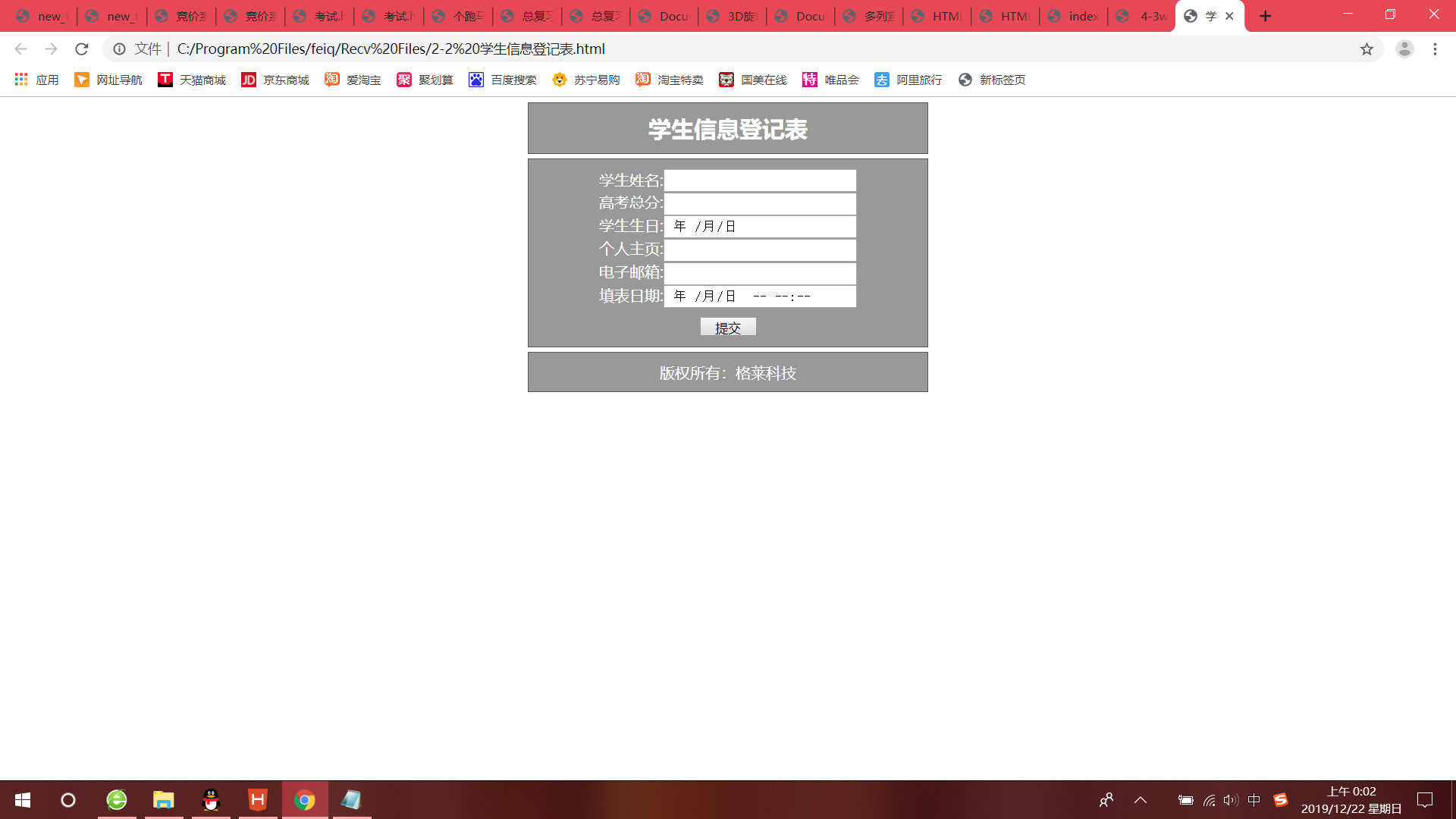<!DOCTYPE html>
<html lang="zh">

<head>
<meta charset="UTF-8" />
<title>学生信息登记表</title>
<style>
* {
padding: 0;
margin: 0;
}

ul,
li {
list-style: none;
}

header,
nav,
main,
footer {
width: 400px;
margin: 5px auto 5px;
border: 1px solid #555;
background: #999;
padding: 10px;
color: #fff;
text-align: center;
}

input {
width: 200px;
height: 20px;
}

#submit {
width: 60px;
height: 20px;
margin-top: 10px;
}
</style>
</head>
<body>

<header>
<h2>学生信息登记表</h2>
</header>

<main>
<form action="" method="post">
<label for="">学生姓名:</label><input type="text" id="name" /><br />
<label for="">高考总分:</label><input type="number" id="score" max="750" min="0" /><br />
<label for="">学生生日:</label><input type="date" id="birthday" /><br />
<label for="">个人主页:</label><input type="url" id="web" /><br />
<label for="">电子邮箱:</label><input type="email" id="mail" /><br />
<label for="">填表日期</label>:<input type="datetime-local" id="data" /><br />
<input type="submit" id="submit" value="提交" />
</form>
</main>
<footer>版权所有：格莱科技</footer>

</body>
<script type="text/javascript">
window.onload = function() {
var submit = document.getElementById("submit"); //获取提交按钮
submit.onclick = function() { //为提交按钮添加点击事件
var name = document.getElementById("name"); //获取学生姓名
var score = document.getElementById("score"); //获取高考总分
var birthday = document.getElementById("birthday"); //获取学生生日
var web = document.getElementById("web"); //获取个人主页
var mail = document.getElementById("mail"); //获取电子邮箱
var data = document.getElementById("data"); //获取填表日期
if(name.value.length <= 1 || name.value.length >= 7) { //判断明星长度是否为2-6位
alert("姓名请输入2-6个字符"); //弹出错误提示
return false; //禁止表单提交

} else if(birthday.value == '') {
alert("请填写学生生日");
return false;
} else if(web.value == '') {
alert("请填写个人主页");
return false;
} else if(mail.value == '') {
alert("请填写电子邮箱");
return false;
} else if(data.value == '') {
alert("请填写填表日期");
return false;
}
}
}
</script>

</html>



展开全文• case 2: if(flag==0){ cout请先选择1，建立学生信息后再操作"; system("pause"); system("cls"); goto t; } system("cls"); cout显示学生3门课程总分"; printfallscore(head); system("pause");system("cls");goto ...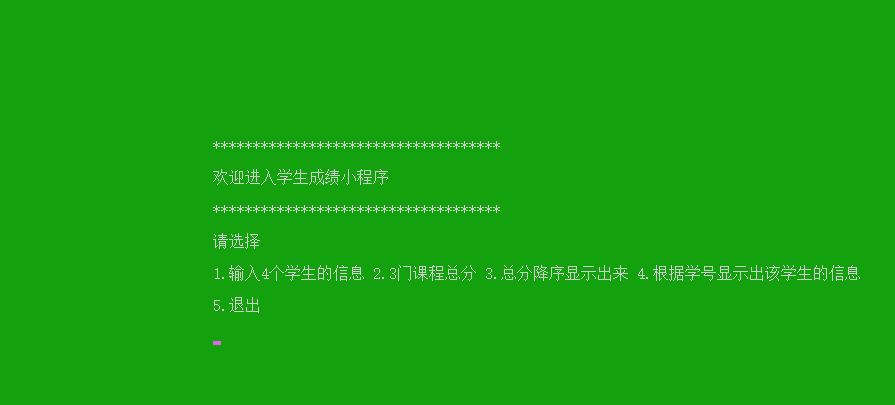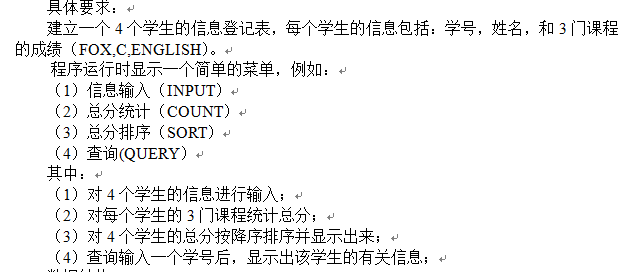#include <iostream>
#include <windows.h>
#include <bits/stdc++.h>
using namespace std;
typedef struct student
{
long int num;//学号
char name;//姓名
int foxscore;//fox成绩
int cscore;//C语言
int englishscore;//英语成绩
int allscore;//总成绩
struct student *next;
}stu,*st;

void goxy(int x,int y)
{

HANDLE handle = GetStdHandle(STD_OUTPUT_HANDLE);
COORD pos = { 0 };
pos.X = x;
pos.Y = y;
SetConsoleCursorPosition(handle, pos);
}
void setcolor(unsigned short ForeColor,unsigned short BackGroundColor)
{
HANDLE handle=GetStdHandle(STD_OUTPUT_HANDLE);
SetConsoleTextAttribute(handle,ForeColor+BackGroundColor*0x10);
}
void caidan()
{

goxy(30,10);
printf("************************************");
goxy(30,12);
printf("欢迎进入学生成绩小程序");
goxy(30,14);
printf("************************************");
goxy(30,16);
printf("请选择");
goxy(30,18);
printf("1.输入4个学生的信息 2.3门课程总分 3.总分降序显示出来 4.根据学号显示出该学生的信息");
goxy(30,20);
printf("5.退出");
goxy(30,22);
}
void getinformation(st &head){
st qhead;
qhead=head;
for(int i=1;i<5;i++){
st p=new stu;
p->next=NULL;
cin>>p->num>>p->name>>p->foxscore>>p->cscore>>p->englishscore;
p->allscore=p->cscore+p->foxscore+p->englishscore;
qhead->next=p;
qhead=p;
}
}
void printfallscore(st head){
st p=head->next;
cout<<"4名同学的总成绩如下"<<endl;
while(p){
cout<<p->name<<" "<<p->allscore<<endl;
p=p->next;
}
}

st charu(st head,st &q){
st p1,p2;
p1=head;
p2=head->next;

while(p2!=NULL){
if(q->allscore<p2->allscore){
p1=p1->next;
p2=p2->next;
}else break;
}
p1->next=q;
q->next=p2;

return head;

}
st mysort(st head){
st p1,q,t;
p1=head;

q=head->next->next;
p1->next->next=NULL;

t=q->next;

while(q!=NULL){
p1=charu(p1,q);
q=t;
if(t!=NULL)
t=q->next;
}
return p1;

}
void searchnum(st head){
cout<<"请输入要查找的学号"<<endl;
int x,y=0;
cin>>x;
st p;
p=head->next;
while(p){
if(p->num==x){
cout<<p->num<<" "<<p->name<<" "<<p->foxscore<<" "<<p->cscore<<" "<<p->englishscore;
y=1;
break;
}
p=p->next;
}
if(y==0) cout<<"没有要找的学号"<<endl;

}
int main()
{
st head=new stu;
head->next=NULL;
//cout << "对4个学生的信息进行输入；" << endl;
//getinformation(head);//1 a 10 20 30 2 b 20 30 40 3 c 45 50 60 4 d 70 80 90

setcolor(7,2);
system("cls");

int i,flag=0;
t:caidan();
scanf("%d",&i);
switch (i){
case 1:system("cls");
cout<<"对4个学生的信息进行输入；例如 1 a 10 20 30 2 b 20 30 40 3 c 45 50 60 4 d 70 80 90"<<endl;
getinformation(head);//1 a 10 20 30 2 b 20 30 40 3 c 45 50 60 4 d 70 80 90//10 fu 99 100 100 15 zhao  80 70 60 20 qian 15 25 35 30 wang 55 56 57
flag=1;
system("pause");system("cls");goto t; break;
case 2:
if(flag==0){
cout<<"请先选择1，建立学生信息后再操作"<<endl;
system("pause");
system("cls");
goto t;
}
system("cls");
cout<<"显示学生3门课程总分"<<endl;
printfallscore(head);
system("pause");system("cls");goto t;break;
case 3:
if(flag==0){
cout<<"请先选择1，建立学生信息后再操作"<<endl;
system("pause");
system("cls");
goto t;
}
system("cls");
cout<<"对4个学生的总分按降序排序并显示出来"<<endl;
head=mysort(head);
printfallscore(head);
system("pause");system("cls");goto t;break;
case 4:
if(flag==0){
cout<<"请先选择1，建立学生信息后再操作"<<endl;
system("pause");
system("cls");
goto t;
}
cout<<"输入一个学号，显示出该学生的有关信息"<<endl;
searchnum(head);
system("pause");system("cls");goto t;break;
case 5:exit(1);break;
default:system("cls");goto t;
}

return 0;
}




展开全文数据结构
• C++实现学生成绩信息登记表 学生成绩信息登记表： 每个学生的数据包括：学号，姓名，和语数英三门的成绩 实现效果： 1.从键盘输入3名学生的数据 2.显示每个学生的三门课的平均分 3.显示每门课的全班平均分 4.按平均...
C++实现学生成绩信息登记表
学生成绩信息登记表：
每个学生的数据包括：学号，姓名，和语数英三门的成绩
实现效果：
1.从键盘输入3名学生的数据
2.显示每个学生的三门课的平均分
3.显示每门课的全班平均分
4.按平均分高低排名，并按名次降序输出学生的所有数据
//学生成绩信息登记表
//每个学生的数据包括：学号，姓名，和语数英三门的成绩
//实现效果：
//1.从键盘输入3名学生的数据
//2.显示每个学生的三门课的平均分
//3.显示每门课的全班平均分
//4.按平均分高低排名，并按名次降序输出学生的所有数据
#include <iostream>
using namespace std;
#define STU 3   //设置学生的人数

//定义学生结构体
struct Student {
int num;//学号
string name;//姓名
int gradeChinese;//语文成绩
int gradeMath;//数学成绩
int gradeEnglish;//英语成绩
};
//得到输入的学生的信息
void getStudentInfo(Student a[STU]) {
for (int i = 0;i < STU;i++)
{
cout << "请输入第" << i + 1 << "个同学的" << "学号:" ;
cin >> a[i].num;
cout << "请输入第" << i + 1 << "个同学的" << "姓名:" ;
cin >> a[i].name;
cout << "请输入第" << i + 1 << "个同学的" << "语文成绩:" ;
cin >> a[i].gradeChinese;
cout << "请输入第" << i + 1 << "个同学的" << "数学成绩:" ;
cin >> a[i].gradeMath;
cout << "请输入第" << i + 1 << "个同学的" << "英语成绩:"  ;
cin >> a[i].gradeEnglish;
}
cout << endl;
}
//计算每个学生的三门平均分
void averageStudent(Student a[STU]) {
for (int i = 0;i < STU;i++)
{
cout << "第" << i + 1 << "名同学的语数英平均分为："
<< (a[i].gradeChinese + a[i].gradeEnglish + a[i].gradeMath) / 3
<< endl;
}
cout << endl;
}

//计算每门课的全班平均分
void averageClass(Student a[STU]) {
int a_math = 0;//全班数学总分
for (int i = 0;i < STU;i++)
{
a_math = a_math + a[i].gradeMath;
}
int a_chinese = 0;//全班语文总分
for (int i = 0;i < STU;i++)
{
a_chinese = a_chinese + a[i].gradeChinese;
}
int a_english = 0;//全班英语总分
for (int i = 0;i < STU;i++)
{
a_english = a_english + a[i].gradeEnglish;
}
cout << "全班语文的平均分为："<<a_chinese/STU<<endl;
cout << "全班数学的平均分为："<<a_math/STU<<endl;
cout << "全班英语的平均分为："<<a_english/STU<<endl;
cout << endl;
}

//按平均分降序排名
void rankStudentGrade(Student a[STU]) {
int b[STU];//定义b数组存放每个人的平均分
for (int i = 0;i < STU;i++)
{
b[i] = (a[i].gradeChinese + a[i].gradeEnglish + a[i].gradeMath) / 3;
}
//把b数组数据存一份数据给c数组
//b数组中存放的是未排序的平均分，c数组中存放的是排序过后的平均分
int c[STU];
for (int i = 0;i < STU;i++)
{
c[i] = b[i];
}
//冒泡排序
for (int i = 0;i < STU - 1;i++)
{
for (int j = 0;j < STU - 1 - i;j++)
{
int temp = 0;
if (c[j] < c[j + 1])
{
temp = c[j + 1];
c[j + 1] = c[j];
c[j] = temp;
}
}
}
//测试输出
//for (int i = 0;i < STU;i++)
//{
//	cout << "按平均分降序排名为：" << c[i] << endl;
//}

//比较b和c数组，输出对应平均分同学的成绩
for (int i = 0;i < STU;i++)
{
for (int j = 0;j < STU;j++)
{
if (c[i] == b[j])
{
cout << "第" << i + 1 << "名是：" << a[j].name << "  平均分为：" << b[j]
<<"  学号为："<<a[j].num
<< "  语文成绩为：" << a[j].gradeChinese
<< "  数学成绩为：" << a[j].gradeMath
<< "  英语成绩为：" << a[j].gradeEnglish
<< endl;
}
}
}

}

int main()
{
Student a[STU];

getStudentInfo(a);//得到输入的学生的信息

//cout << "第三位同学的英语成绩为：" << a[STU - 1].gradeEnglish << endl;//测试是否接收成功

averageStudent(a);//计算每个学生的三门平均分

averageClass(a);//计算每门课的全班平均分

rankStudentGrade(a);//按平均分降序排名

system("pause");
return 0;

}--------------------------降序输出算法改进-----------------------------
//学生成绩信息登记表
//每个学生的数据包括：学号，姓名，和语数英三门的成绩
//实现效果：
//1.从键盘输入3名学生的数据
//2.显示每个学生的三门课的平均分
//3.显示每门课的全班平均分
//4.按平均分高低排名，并按名次降序输出学生的所有数据
#include <iostream>
using namespace std;
#define STU 3   //设置学生的人数

//定义学生结构体
struct Student {
int num;//学号
string name;//姓名
int gradeChinese;//语文成绩
int gradeMath;//数学成绩
int gradeEnglish;//英语成绩
};
//得到输入的学生的信息
void getStudentInfo(Student a[STU]) {
for (int i = 0;i < STU;i++)
{
cout << "请输入第" << i + 1 << "个同学的" << "学号:" ;
cin >> a[i].num;
cout << "请输入第" << i + 1 << "个同学的" << "姓名:" ;
cin >> a[i].name;
cout << "请输入第" << i + 1 << "个同学的" << "语文成绩:" ;
cin >> a[i].gradeChinese;
cout << "请输入第" << i + 1 << "个同学的" << "数学成绩:" ;
cin >> a[i].gradeMath;
cout << "请输入第" << i + 1 << "个同学的" << "英语成绩:"  ;
cin >> a[i].gradeEnglish;
}
cout << endl;
}
//计算每个学生的三门平均分
void averageStudent(Student a[STU]) {
for (int i = 0;i < STU;i++)
{
cout << "第" << i + 1 << "名同学的语数英平均分为："
<< (a[i].gradeChinese + a[i].gradeEnglish + a[i].gradeMath) / 3
<< endl;
}
cout << endl;
}

//计算每门课的全班平均分
void averageClass(Student a[STU]) {
int a_math = 0;//全班数学总分
for (int i = 0;i < STU;i++)
{
a_math = a_math + a[i].gradeMath;
}
int a_chinese = 0;//全班语文总分
for (int i = 0;i < STU;i++)
{
a_chinese = a_chinese + a[i].gradeChinese;
}
int a_english = 0;//全班英语总分
for (int i = 0;i < STU;i++)
{
a_english = a_english + a[i].gradeEnglish;
}
cout << "全班语文的平均分为："<<a_chinese/STU<<endl;
cout << "全班数学的平均分为："<<a_math/STU<<endl;
cout << "全班英语的平均分为："<<a_english/STU<<endl;
cout << endl;
}

//按平均分降序排名
void rankStudentGrade(Student a[STU]) {
int b[STU];//定义b数组存放每个人的平均分
for (int i = 0;i < STU;i++)
{
b[i] = (a[i].gradeChinese + a[i].gradeEnglish + a[i].gradeMath) / 3;
}

for (int i = 0;i < STU - 1;i++)
{

for (int j = 0;j < STU - 1 - i;  j++)
{
if (b[j] < b[j+1])
{
Student temp;
temp = a[j];
a[j] = a[j+1];
a[j+1] = temp;

int temp1 = 0;
temp1 = b[j];
b[j] = b[j + 1];
b[j + 1] = temp1;

}
}
}

for (int j = 0;j < STU;j++)
{
cout << "第" << j + 1 << "名是：" << a[j].name
<<"平均分为："<< (a[j].gradeChinese + a[j].gradeEnglish + a[j].gradeMath) / 3
<< "  学号为：" << a[j].num
<< "  语文成绩为：" << a[j].gradeChinese
<< "  数学成绩为：" << a[j].gradeMath
<< "  英语成绩为：" << a[j].gradeEnglish
<< endl;
}
}

int main()
{
Student a[STU];

getStudentInfo(a);//得到输入的学生的信息

//cout << "第三位同学的英语成绩为：" << a[STU - 1].gradeEnglish << endl;//测试是否接收成功

averageStudent(a);//计算每个学生的三门平均分

averageClass(a);//计算每门课的全班平均分

rankStudentGrade(a);//按平均分降序排名

system("pause");
return 0;

}


转载自：黑凤梨の博客


展开全文c++
•表格模板
• 2013年中小学生学籍管理全国联网，学生学籍信息采集校对工作量很大，“学生学籍信息登记表批量打印”软件可以打印带有学生照片的校对表，操作简便，能很好提高数据校对效率和准确性。
• 数据库以及的创建 *对于数据库张字段的数据类型的使用，方便数据在输入的时候不会出错，进而经常会使用varchar类型 步骤二 利用winform窗体的进行相关控件的使用 *主要控件的使用DateGridView控件 DateGridView...
步骤一
数据库以及表的创建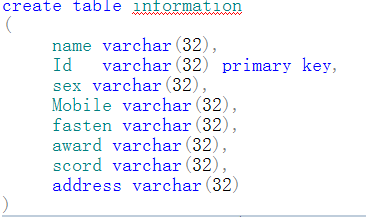*对于数据库张字段的数据类型的使用，方便数据在输入的时候不会出错，进而经常会使用varchar类型
*对于涉及到的数据库连接对单独放到可配置文件当中
即app文件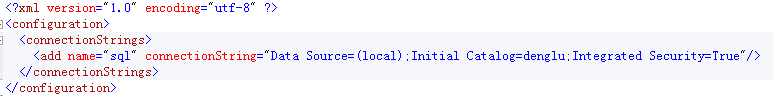步骤二
利用winform窗体的进行相关控件的使用
*主要控件的使用DateGridView控件
DateGridView控件的相关的属性：row 、cells、
DateGridView控件的相关的事件：cellsClick
DateGridView控件的相关的类：DateGridViewRow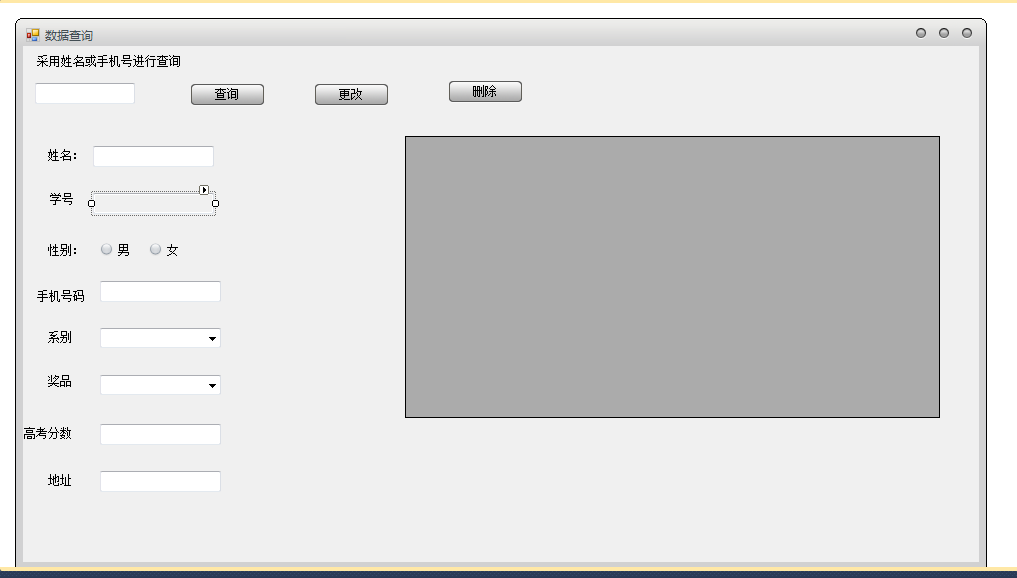步骤三
在vs中进行编码的编写
using System;
using System.Collections.Generic;
using System.ComponentModel;
using System.Data;
using System.Drawing;
using System.Linq;
using System.Text;
using System.Windows.Forms;
using System.Data.SqlClient;
using System.Configuration;
namespace xiangmu
{
public partial class 数据查询 : Form
{
public 数据查询()
{
InitializeComponent();
}
private void Date()
{
#region   对数据库中的数据进行查询
//创建可配置文件，进行存放数据库的连接
string sql = ConfigurationManager.ConnectionStrings["sql"].ConnectionString;
//应用SqlConnection类建立连接的对象
SqlConnection conn = new SqlConnection(sql);
//打开数据库
conn.Open();
//应用SqlCommand类建立命令对象
//三种格式创建命令对象
SqlCommand comm = conn.CreateCommand();
comm.CommandText = "select *from information where
name=@name or Mobile
=@Mobile ";
/*SqlCommand comm=new SqlCommand();
comm.Connection = conn;
comm.CommandText = "select *from information where
name=@name or Mobile
=@Mobile ";*/
/*SqlCommand comm=new SqlCommand("select *from information where
name=@name or Mobile
=@Mobile",conn);*/
comm.Parameters.AddWithValue("@name", textBox1.Text.ToString());
comm.Parameters.AddWithValue("@Mobile", textBox1.Text.ToString());
//应用数据适配器创建对象，执行数据库语句，获得相关的数据
SqlDataAdapter adapter = new SqlDataAdapter(comm);
//应用数据表类，创建对象，进行数据库的数据的存放
DataTable data = new DataTable();
//将从数据库获得信息放置在数据表类中的一个对象当中，实现从数据库中显示在vs中
adapter.Fill(data);
this.dataGridView1.DataSource = data;
//数据库连接的关闭
conn.Close();
//命令的释放
comm.Dispose();
//连接的释放
#endregion
}
private void button1_Click(object sender, EventArgs e)
{
//对数据查询函数的调用
Date();
}
private void dataGridView1_CellClick(object sender, DataGridViewCellEventArgs e)
{
#region 从 DataGridView控件中获得相关的信息
if (this.dataGridView1.SelectedRows.Count > 0)
{
//应用 DataGridViewRow 类创建他的对象，
DataGridViewRow row = this.dataGridView1.SelectedRows;

//从 DataGridView控件中获得相关的信息
this.textBox2.Text = row.Cells.Value.ToString();
this.textBox6.Text = row.Cells.Value.ToString();
string gender = row.Cells.Value.ToString();
if (gender == "男")
{
radioButton1.Checked = true;
}
if (gender == "女")
{
radioButton2.Checked = true;
}
this.textBox3.Text = row.Cells.Value.ToString();
this.comboBox1.Text = "";
this.comboBox1.Text = row.Cells.Value.ToString();
this.comboBox2.Text = "";
this.comboBox2.Text = row.Cells.Value.ToString();
this.textBox4.Text = row.Cells.Value.ToString();
this.textBox5.Text = row.Cells.Value.ToString();
#endregion
}
}
private void button2_Click(object sender, EventArgs e)
{
#region   对数据中的数据进行更改
string sql = ConfigurationManager.ConnectionStrings["sql"].ConnectionString;
SqlConnection conn = new SqlConnection(sql);
conn.Open();
SqlCommand comm = conn.CreateCommand();
string id = textBox1.Text;
//对数据进行更改
comm.CommandText = "update information set
name=@name, sex=@sex ,Mobile=@Mobile,fasten=@fasten ,award=@award ,scord=@scord ,address=@address whereId=@id
";
comm.Parameters.AddWithValue("@id", textBox6.Text.ToString());
comm.Parameters.AddWithValue("@name", textBox2.Text.ToString());
if (radioButton1.Checked == true)
{
comm.Parameters.AddWithValue("@sex", radioButton1.Text.ToString());
}
if (radioButton2.Checked == true)
{
comm.Parameters.AddWithValue("@sex", radioButton2.Text.ToString());
}
comm.Parameters.AddWithValue("@Mobile", textBox3.Text.ToString());
comm.Parameters.AddWithValue("@fasten", comboBox1.Text.ToString());
comm.Parameters.AddWithValue("@award", comboBox2.Text.ToString());
comm.Parameters.AddWithValue("@scord ", textBox4.Text.ToString());
comm.Parameters.AddWithValue("@address", textBox5.Text.ToString());
comm.ExecuteNonQuery();
MessageBox.Show("更改成功");
Date();
#endregion
}
private void button3_Click(object sender, EventArgs e)
{
#region 对数据库中的数据进行删除
DataGridViewRow row = this.dataGridView1.SelectedRows;
string sql = "Data Source=(local);Initial Catalog=denglu;Integrated Security=True";
SqlConnection conn = new SqlConnection(sql);
conn.Open();
SqlCommand comm = conn.CreateCommand();
string id = textBox1.Text;
comm.CommandText = "delete from  information where
Id=@Id";
comm.Parameters.AddWithValue("@Id", row.Cells.Value.ToString());
comm.ExecuteNonQuery();
comm.Dispose();
conn.Close();
conn.Dispose();
MessageBox.Show("删除成功");
textBox2.Text = "";
textBox6.Text = "";
textBox3.Text = "";
comboBox1.Text = "";
comboBox2.Text = "";
textBox4.Text = "";
textBox5.Text = "";
//获取当前选中的行，返回值为DataGridViewRow
this.dataGridView1.Rows.Remove(row);
#endregion
}
}
}
在很多时候坐在电脑面前编写代码，对一个小程序的思考后，按照自己的思路将效果实现，这里的思考，有时我们会把问题思考的变得稍微难一些。其结果，我们可以看到几行代码就可以让我们看到很好的有趣的程序的诞生，所以，我们对于编程，用简单的代码编写最完美的程序。


展开全文c# winform
• {管理信息化 ERPMRP}ERP 手工沙 盘学生团队运营 起始年 任务清单每执行完一项操作CEO 请在相应的方格 内打勾 新年度规划会议 请按顺序执行下列各项操作财务总监助理 参加订货会/登记销售订单 在方格中填写现金收支...
• 问题 ... 在界面输入学号，点击...如果没有，弹出“学生信息不存在”提示信息。 代码 import javax.swing.*; import java.awt.*; import java.io.FileInputStream; import java.io.FileOutputStream; import java.ut...
• 实现功能输入输出插入删除查找追加读入显示保存拷贝排序索引分类合计退出 能实现对学生信息的简单管理 具体要求 建立一个4个学生的信息登记表每个学生的信息包括学号姓名和3门课程的成绩FOX,C,ENGLISH ?程序运行时...
• 数据库综合实验重做因为期末的时候做数据库综合实验太匆忙，很多地方都是能用就好，做完之后突然想到可以改进的方法，所以现在寒假来重做一下题目如下：设计某高校学生选课管理系统实现学生信息、课程学生管理；...
• 建立一个4个学生信息登记表，每个学生的信息包括：学号、姓名和3门课程的成绩（math、c、english）程序运行时显示一个简单的菜单。例如： a) 信息输入（input） b) 总分统计（count） c) 总分排序（sort） d) 查询...
• 实用标准 学生成绩管理 实现功能输入输出插入删除查找追加读入显示保存 拷贝排序索引分类合计退出 能实现对学生信息的简单管理 具体要求 建立一个 4 个学生的信息登记表每个学生的信息包括学号姓名和 3 门课程的成绩...
• 精品文档 精品文档 PAGE PAGE # 欢迎下载 学生成绩管理 实现功能输入输出插入删除查找追加读入显示保存 拷贝排序索引分类合计退出 能实现对学生信息的简单管理 具体要求 建立一个 4 个学生的信息登记表每个学生的...
• word word 专业资料 学生成绩管理 实现功能输入输出插入删除查找追加读入显示保存拷贝排序索引分类合计退出 能实现对学生信息的简单管理 具体要求 建立一个 4 个学生的信息登记表每个学生的信息包括学号姓名和 3 门...
• 线性表一、线性表的基本概率1、线性表的定义2、线性表举例3、线性表的特点4、线性表的基本操作二、线性表的顺序存储1...例如，学生信息登记表。 在复杂的线性表，常把数据元素称为记录（Record），它由若干个数据项（I数据结构 算法 线性代数 c++ c语言
• 可根据数值进行升序或者降序的排列·比如创建班级的学生信息登记表，可以将学号使用序列自动生成。班级的学号都是10000~10060这个范围，每登记一个学生，序列自动增长一个。1、创建序列的命令...
• D1 学生信息表 D3 课程信息 学生基 本信息 课程信息 新生报到 1.2 1.3 成绩信息 注册 建档分班 课程确定 班级 信息 D2 班级信息 D4 成绩信息 学生 毕业分档 1.4 成绩信息 处理档案 系统的主要...
• 修改已知学号的学生信息 删除已知学号的学生信息 查找已知学号的学生信息 按学号，专业输出学生籍贯 查询学生学籍变化，比如入学，转专业，退学，降级，休学，毕业 源码如下： #include<stdio.h> #include...编程语言 C语言 C语言课程设计
• 1· 要做一个信息管理系统，首先这个系统要方便操作，登录到系统后信息一目了然2·1）用自定义结构体设计该系统的数据连接 2）用数组或指针链表将所有学生的数据按学号顺序链接起来 3）程序能够显示已经输入的信息3....
• /* * name:Student_information.cpp * author:xdc * time:2013年3月31日15:58:58 * * 问题描述: ... * ⑴ 插入：将某学生的基本信息插入到登记表中； * ⑵ 删除：将满足条件的基本信息删除； * ⑶ 修
• ④. 系统数据库至少应包含数据对象：学生表、选课表（学生只对对培养方案中的选修课有选课权）、课程表（可参照本学校实际执行的课程表表进行设计）、成绩登记表（可参照本学校成绩登记表进行设计）、培养方案、教师...
• 理的需要具有对产品员工客户管理的功能销售员可以对销售的产品进行登记并 将销售情况反馈给数据库管理员可以查看员工信息以及销售情况管理员登陆后可以添 加删除员工以及销售员以外的特殊操作为管理的方便性和信息...
• 高中学生的高考档案，主要有4-5样文件：《2020年浙江省普通高校招生考试考生报名登记表》、《浙江省普通高校招生体格检查表》、《浙江省普通高中毕业生登记表》、《浙江省普通高中毕业生综合素质信息表》。部分学生...
• 但在实际的编程过程中，我们往往还需要一组类型不同的数据，例如对于学生信息登记表，姓名为字符串，学号为整数，年龄为整数，所在的学习小组为字符，成绩为小数，因为数据类型不同，显然不能用一个数组来存放。...
• 审批通过后到国家制定网站登记贷款 * 修改密码（若忘记密码，只能通过管理员修改） 后台（管理员使用） * 系统管理 * 权限分配： * 超级管理员：（权限代码：0） * 维护系统基础数据（学院、宿舍、党务、...
• 目前高校学生上课考勤管理都是以任课老师上课点名，记录学生上课出勤情况，学期末根据上课出勤表及作业登记表学生平时成绩打分，然后把数据上交到各院系。学生请假以传统的写请假条的形式向各自班主任请假，时间长...
• 逐个查询一、信息查询界面往往在登记学生成绩的时候我们的工作是以班级命名的，如果要查询某个学生的成绩在使用函数公式可能会比较麻烦一些！今天的我们的查询案例中只是要根据学号进行跨查询并且还要查到他......